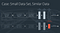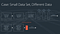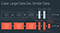# PyTorch Deep Learning Nanodegree: Convolutional Neural Networks

A third part of the Nanodegree: CNN

Introduction

Neural Networks

Convolutional Neural Networks

Recurrent Neural Networks

Deploying a Model

The end of this journey

# General

In this lesson we learn about convolutional neural nets, try transfer learning and style transfer, understand the importance of weight initialization, train autoencoders and do many other things. Also, we’ll work on a second project — Dog-Breed Classifier.

## Convolutional Neural Nets

In this lesson, we go learn more about neural nets: writing MLP and their comparison to CNN, how CNN work, image augmentations, and other things. I’ll skip this section. Why? Because it is available and free to anyone. There is a free course by Udacity: Introduction to Neural Networks. Lesson 5 of this course contains this lesson, so you can go through it if you are interested.

## Transfer Learning

Transfer learning is widely used in a lot of computer vision problems, for example in image classification and segmentation. Basically, it works like this: someone trains a deep CNN on ImageNet or some other big dataset with images and makes it available for public usage. Now we can use this model with pre-trained weights and apply it for our tasks on a smaller dataset and the quality of the model will usually be higher than if we tried to train a new model from scratch.

Transfer Learning — video with a short description of transfer learning.

Useful Layers — more information on layers with pre-trained weights.

Fine-Tuning — how to use pre-trained neural nets.

There are four main cases depending on our dataset:

1. New data set is small, new data is similar to original training data.
2. New data set is small, new data is different from original training data.
3. New data set is large, new data is similar to original training data.
4. New data set is large, new data is different from original training data.

New data set is small, new data is similar to original training data.

• slice off the end of the neural network
• add a new fully-connected layer that matches the number of classes in the new data set
• randomize the weights of the new fully connected layer; freeze all the weights from the pre-trained network
• train the network to update the weights of the new fully connected layerAdding and training a fully-connected layer at the end of the NN.

As the new data is smaller and similar to the original data, we can keep most of the pre-trained net and train only the newly added last dense layer.

Small Data Set, Different Data

• slice off all but some of the pre-trained layers near the beginning of the network
• add to the remaining pre-trained layers a new fully-connected layer that matches the number of classes in the new data set
• randomize the weights of the new fully connected layer; freeze all the weights from the pre-trained network
• train the network to update the weights of the new fully connected layerRemove all but the starting layers of the model, and add and train a linear layer at the end.

As we have different data, we can’t use the whole pre-trained net — its last layers were trained to look for different features, so it may work not well on our data. And if we keep only several top layers (which recognize more general features), our model would be able to learn features relevant to our dataset.

Large Data Set, Similar Data

• remove the last fully connected layer and replace with a layer matching the number of classes in the new data set
• randomly initialize the weights in the new fully connected layer
• initialize the rest of the weights using the pre-trained weights
• re-train the entire neural networkUtilizing pre-trained weights as a starting point!

The idea is that we won’t overfit if we train the whole net on a large dataset.

Large Data Set, Different Data

• remove the last fully connected layer and replace with a layer matching the number of classes in the new data set
• retrain the network from scratch with randomly initialized weights
• alternatively, you could just use the same strategy as the “large and similar” data case

The idea is that if we have a different dataset than we would need to train our own net.

VGG Model & Classifier — a practical video.

Freezing Weights & Last Layer

Training a Classifier

## Weight Initialization

Weight initialization is really important. Bad initial weights could make neural net unable to train, so a good initialization method should be used.

Weight Initialization

Constant Weights — what would happen if we set constant weights?

Random Uniform

General Rule

Normal Distribution — weight initialization with normal distribution

Solution and Default Initialization — solution for exercise notebook on weight initialization. The code is available here.

## Autoencoders

In this lesson, we learn about autoencoders, which can compress the data into a smaller feature space and then turn it back into original dimensions. The code is available here: https://github.com/udacity/deep-learning-v2-pytorch/tree/master/autoencoder

Autoencoders — general information

The simplest form of autoencoders is linear autoencoder. We will learn to use in to compress digits in MNIST dataset.

Defining & Training an Autoencoder

A Simple Solution

Learnable Upsampling — CNN are obviously better at upsampling than linear models.

Transpose Convolutions

Convolutional Autoencoders use convolutional layers and are better than linear.

Convolutional Autoencoder

Convolutional Solution

Upsampling & Denoising

Denoising autoencoders add random noise to the input data so that the net generalizes better.

De-noising

## Style Transfer

As I wrote in my first blog post in the series, style transfer allows you to take one image and transfer its style to some other image.

This lesson is also available in the free course by Udacity: Introduction to Neural Networks. Lesson 6 of this course contains this lesson, so you can go through it if you are interested. You can find the code here: https://github.com/udacity/deep-learning-v2-pytorch/tree/master/style-transfer

## Project: Dog-Breed Classifier

This is the second project of this course. In this project, we will try to build an algorithm which will at first decide whether an image contains a dog or a human and then it will predict the dog’s breed. The code template for this project can be found here: https://github.com/udacity/deep-learning-v2-pytorch/tree/master/project-dog-classification

Let’s follow the project’s steps! We have two datasets: 13233 human photos and 8351 dog images.

Detect human faces

We have a code for face detection using OpenCV — simple Haar feature-based cascade classifier. It works okay, but not very well as you can see below:

So I have decided to use a different approach and used the code from this repository: https://github.com/TropComplique/mtcnn-pytorch

It uses Multi-task Cascaded Convolutional Networks to detect faces and the result is better. But there were many false positives on dog images. I suppose the reason is that the model is tailored to human faces and searches for them even when there are none.

Detecting dogs

At first, we use a baseline approach: we simply use a pre-trained VGG16 model to make predictions. Here is a nice trick: you can see all 1000 ImageNet classes here. Keys 151–268 describe dog breeds, so if VGG16 return prediction in this range, we can say that a dog was detected. And this works great!

I was interested in trying other approaches and used pre-trained ResNet-18, the results were even better:

Train CNN on dog breeds from scratch

Next step is training our own model. At first, we train it from scratch (as an exercise).

I wrote my custom Dataset class:

`class DogsDataset(Dataset):    def __init__(self, datafolder, transform = transforms.Compose([transforms.CenterCrop(32),transforms.ToTensor()])):        self.datafolder = datafolder        self.image_files_list = []        self.transform = transform        self.labels = []        for folder in glob(datafolder + '/*'):            for img in glob(folder + '/*'):                self.labels.append(int(folder.split('.')[-3:]))                self.image_files_list.append(img)                    def __len__(self):        return len(self.image_files_list)def __getitem__(self, idx):        img_name = self.image_files_list[idx]                image = Image.open(img_name).convert('RGB')        image = self.transform(image)                label = self.labels[idx]        return image, label`

And a multi-layer CNN:

`class Net(nn.Module):    def __init__(self):        super(Net, self).__init__()        self.conv1 = nn.Conv2d(3, 16, 3, stride=2, padding=1)        self.conv2 = nn.Conv2d(16, 32, 3, stride=2, padding=1)        self.conv3 = nn.Conv2d(32, 64, 3)        self.pool = nn.MaxPool2d(2, 2)        self.fc1 = nn.Linear(2304, 512)        self.fc3 = nn.Linear(512, 133)        self.dropout = nn.Dropout(0.1)def forward(self, x):        x = self.pool(F.relu(self.conv1(x)))        x = self.pool(F.relu(self.conv2(x)))        x = self.pool(F.relu(self.conv3(x)))        x = x.view(-1, 2304)        x = self.dropout(x)        x = F.relu(self.fc1(x))        x = self.dropout(x)        x = self.fc3(x)        return x`

It achieved a test accuracy of 12% (104 images of 836) which was enough to pass this task.

Train CNN using transfer learning

In this section I made a baseline using ResNet-18 again:

`model_transfer = models.resnet18(pretrained=True)for param in model_transfer.parameters():    param.requires_grad = Falsemodel_transfer.fc = nn.Linear(512, 133)`

Obviously, it worked much better, achieving an accuracy of 74% (623/836).

Writing the final algorithm

And now we wrote an algorithm with the following logic:

• if a dog is detected in the image, return the predicted breed.
• if a human is detected in the image, return the resembling dog breed.
• if neither is detected in the image, provide an output that indicates an error.

Here are some results:

The algorithm didn’t work very well, I suppose the main reason is small dataset and simple backbone for CNN model. If we had a better model and more data, the results would be better. I suppose this is relevant for any Deep Learning project :)

## Deep Learning for Cancer Detection

In this lesson, Sebastian Thrun teaches us about his groundbreaking work on detecting skin cancer with convolutional neural networks.

Intro

Skin Cancer

Survival Probability of Skin Cancer

Medical Classification

The data

Image Challenges

It is really difficult to find skin cancer:

Training the Neural Network

Random vs Pre-initialized Weight

Validating the Training

Sensitivity and Specificity

Diagnosing Cancer

Refresh on ROC Curves

ROC Curve

Comparing our Results with Doctors

Visualization

What is the network looking at?

Refresh on Confusion Matrices

Confusion Matrix

Conclusion

## Jobs in Deep Learning

In this short section, we get a lot of practical ideas on working in deep learning.

How to break into Deep Learning industry?

It is important to look for opportunities and showcase your own skills!

• do job research and see what other skills are required to be successful;
• develop your own app/product: this will prove your skills;
• read recent papers and write your own implementations;

You’d need to master many skills which aren’t present in this Nanodegree, here are some examples:

• Exploring domains such as computer vision, natural language processing, and/or deep reinforcement learning through our other School of AI Nanodegree programs or other resources
• Advancing your programming competency in C++ (a useful language for working with hardware)
• Learning how to build networks in both PyTorch and TensorFlow
• Working with SQL and applying data analysis skills, specifically, how can you clean data or work with very small or large datasets

What do typical deep learning engineers do in their day-to-day

Job-specific tasks differ from company to company, but here are some examples:

• Design and build machine intelligence features
• Develop machine learning algorithms related to deep learning, such as object detection, language translation, and image retrieval in search algorithms
• Deploy analytics models in production and evaluate their scalability
• Code in C++ and Python
• Use ML frameworks such as PyTorch and Tensorflow to implement and prototype deep learning models
• Monitor and update a model after it has been deployed to production
• Employ data augmentation to work with small datasets
• Collaborate with other data and engineering teams on hardware, software architecture hardware, and quality assurance

This was the second part of Deep Learning Nanodegree. We learned how to write CNN and use them for a variety of tasks. Next part will be about Recurrent Neural Nets: RNN, LSTM, word embeddings and more!

Written by

## Data Driven Investor

#### from confusion to clarity, not insanity

Welcome to a place where words matter. On Medium, smart voices and original ideas take center stage - with no ads in sight. Watch
Follow all the topics you care about, and we’ll deliver the best stories for you to your homepage and inbox. Explore
Get unlimited access to the best stories on Medium — and support writers while you’re at it. Just \$5/month. Upgrade